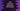# Dart while loop explanation with example# While loop in dart :

Loops are used to run a piece of code for a finite amount of time. For loop and while loop are two mostly used loops. The syntax and idea of both of these loops are the same in almost all programming languages. while loop checks one condition and executes a block of code. It always checks the condition before starting the execution. It will keep running until the condition is true.

## Syntax of Dart while loop :

The syntax of while loop is as below :

``````while (condition){
// code-block
}``````

It checks the condition, if it is true it runs the code-block. It checks the condition again. Note that you need to write some logic in the code-block that makes the condition false at some point of time. Else, it will keep running continuously forever and your program will stop responding.

## Example 1 : Print all numbers from 1 to n :

The below program will print all numbers from 1 to n using one while loop. We are taking the value of n as input from the user.

``````import 'dart:io';

main() {
print("Enter a number : ");
var i = 1;
while (i <= n) {
print(i);
i++;
}
}``````

### Sample Output :

``````Enter a number :
7
1
2
3
4
5
6
7``````

### Explanation :

Here, we have one condition : i<= n. The value of i is incremented on each execution of the code block. Once it will be greater than n, the loop will stop.

## Example 2: Find factorial using while loop :

``````import 'dart:io';

main() {
print("Enter a number : ");